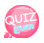# variable-variables

It's too hard to say variable variables.
In short, a variable variable can be used as a variable name.
Some of you may be talking about something.
Consider the following code.

`\$var = "disney";`

In the code above, the variable name is var and the value of the variable is disney.
In the above, the variable value can be used as the variable name.
In other words, the variable disney can be used as a variable name.

## How to use variable variables

`\${"Variable name"}`

자, 아래의 코드를 봅시다.

```\$var = "disney";
\$disney = "var";```

Looking at the code above, I assigned var to the value of the variable disney.
In other words, what value is printed if you print \${"var"} here? The disney variable value var is output.
Look at the following code to see the results.

```<?php
\$var = "disney";
\$disney = "var";
echo \${"var"};
?>
```

Here is the result of the above code:There is one more way to use variable variables. I use \$\$. Consider the following code.

```\$char = "mickey";
\$\$char = "mouse";```

In the code above I assigned the value mickey to the variable char.
Then, oddly, you spend \$twice? \$\$char What is this? I will use the value of the variable char as the variable name. In other words, \$\$char is equivalent to \$mickey.
So \$\$char = "mouse" is equivalent to \$mickey = "mouse".
So let's check the value with an example.

```<?php
\$char = "mickey";
\$\$char = "mouse";

echo \$char;
echo \$mickey
?>```

Here is the result of the above code:Also, \$mickey can be expressed as \${\$char} in the code above.

```<?php
\$char = "mickey";
\$\$char = "mouse";

echo \$char;
echo \${\$char}
?>```

Here is the result of the above code:Now, today we learned about variable variables.